﻿ Graph Theory in an Object Oriented Approach

### Graph Theory in an Object Oriented Approach

Dixit Prasanna Kumar, Sahoo Archana, Badajena Tushar Kumar

Journal of Computer Sciences and Applications

## Graph Theory in an Object Oriented Approach

Dixit Prasanna Kumar1,, Sahoo Archana2, Badajena Tushar Kumar3

1Director, Interface Software, Bhubaneswar

2Completed MCA from OUAT, Bhubaneswar

3Completed B.Tech from ITER, Bhubaneswar

### Abstract

Many real world situations can be describe by means of a diagram consisting of set of points connected by lines. Graph theory has many applications in different field. This paper show how various elements involved in graph theory including graph representations using computer system such as object oriented concept.

• Dixit Prasanna Kumar, Sahoo Archana, Badajena Tushar Kumar. Graph Theory in an Object Oriented Approach. Journal of Computer Sciences and Applications. Vol. 3, No. 6, 2015, pp 123-126. http://pubs.sciepub.com/jcsa/3/6/2
• Kumar, Dixit Prasanna, Sahoo Archana, and Badajena Tushar Kumar. "Graph Theory in an Object Oriented Approach." Journal of Computer Sciences and Applications 3.6 (2015): 123-126.
• Kumar, D. P. , Archana, S. , & Kumar, B. T. (2015). Graph Theory in an Object Oriented Approach. Journal of Computer Sciences and Applications, 3(6), 123-126.
• Kumar, Dixit Prasanna, Sahoo Archana, and Badajena Tushar Kumar. "Graph Theory in an Object Oriented Approach." Journal of Computer Sciences and Applications 3, no. 6 (2015): 123-126.

 Import into BibTeX Import into EndNote Import into RefMan Import into RefWorks

1
Prev Next

### 1. Introduction

Graphs can be used to template many situations or problems in the real world, for example: the problem to find the path for a single walk can be modeled using a graph, where vertices represent cities and edges represent the roads, as shown in Figure 1.Download asVeiw figureFigures index
Figure 1. A graph can be used to find the path between the districts

Other examples are:

•  the cities in a country and the streets that connect them;

•  telecommunication networks, like the Internet and the World Wide Web;

•  mathematical relationships like Fibonacci expansions using trees;

•  decisions trees and Bayesian networks;

•  project management, to manage dependencies between tasks;

•  bioinformatics: protein-protein interaction, residue interaction network, gene regulation;

•  electronic circuits: Kirchhoff laws are deeply related with the graph structure of circuits;

### 2. Review of Literature

According to the author the graphs are helps for solving a complex problem. There are many examples like find out the shortest path in a city from one area to another. BFS and DFS are two algorithms which are use for finding a path, without repeat the edge .

2.2. Y. Daniel Liang’s Procedure

According to the author the graph theoretical ideas can develop in 1736, when Leonard Euler published his paper based on the problem of Seven Bridges of Konigsberg . This problem asks whether there is a continuous path that crosses each bridge only once. In graph, different terminologies are present and also their representation.

2.3. Bondy and Murty’s Procedure

According to the author the graph theory has found many applications in engineering and science.So many books have been published such as by Bondy and Murty. In real world situations can be described, diagram consisting of a set of points together with lines joining certain pairs of these points . Bondy and Murty are both stressed the importance of efficient methods of solving problem. Several good algorithms are include and their efficiencies are analyzed.

2.4. Tero Harju’s Procedure

According to the author the graph theory can found in 1736 when Euler solve the Konigsberg bridge problem. Does there exist a walk crossing each of the seven bridges exactly once? There are no standard notations for graph theoretical objects. This is natural, because the names one uses for the objects reflect the applications.

2.5. Keijo Ruohonen’s Procedure

According to the author the graph is formed by vertices and edges connecting the vertices. Each vertex is indicated by a point and each edge by a line joining the points. There are various types of graphs present with own definitions such as simple graph, direct or indirect graph, weighted or unweighted graph and so on .

### 3. Graph Theory

Graph theory is useful in a graph problem, where a vertex can represent regions and the edges represent movement paths, or movement between the regions. In 1736 Leonard Euler was founded graph theory, when he solve the famous Seven Bridges of Konigsberg problem: Does there exist a walk crossing each of the seven bridges of Konigsberg exactly once? The Pregel river surrounding two central islands, as shown in Figure 2(a).

I1 and I2 are two islands, and P1 and P2 are the cities. Euler’s proof that no such path exists.

Proof: Euler First abstracted the city map into the sketch shown in Figure 2(a). Second, he replace city and islands with a dot, called vertex or a node, and each bridge with a line, called an edge, as shown in Figure 2(b). This structure with vertices and edges called graph.Download asVeiw figureFigures index
Figure 2. Seven bridges connected islands and city http://upload.wikimedia.org/wikipedia/com mons/5/5d/Konigsberg_bridges.png

Let start a walk form any vertex, traversing all edges exactly once and return to the starting vertex. Euler proved that for such path to exist, each vertex must have an even number of edges. Therefore, this problem has no solution.

3.1. What is graph?

A graph is a mathematical structure that represents relationships among entities or objects in the real world, or graphs are represented graphically by drawing a dot or circle for vertex and drawing an arc or line between two vertices for edge. For example, the graph is fig 1 represents the roads and their distances among cities.

A graph is consists of a nonempty set of vertices, nodes and a set of edges that connect the vertices. For convenience, we define a graph as G = (V, E). where V represents a set of vertices E represents a set of edges For example, V and E for the graph in Figure 1 are:

V = {“Balangir”, “Sonapur”, “Bauda”, “Phulabani”, “Baragarh”, “Sambalpur”, “Jharasuguda”, “Sundargarh”, “Deogarh”, “Anugul”};

E = {{“Balangir”, “Sonapur”}, {“Sonapur”, “Bauda”}, {“Bauda”, “Phulabani”}, {“Balangir”, “Baragarh”}, { “Baragarh”, “Sambalpur”}, {“Sambalpur”,

“Jharasuguda”}, {“Sambalpur”, “Deogarh”}, {“Deogarh”, “Anugul”}, …};

3.1.1. Classification of graph

A graph is basically two types:

directed graph and undirected.

In a directed graph, each edge has a direction, which indicates that you can move from one vertex to the other through the edge. It is unidirectional in nature as shown in Figure 3(a).

In an undirected graph, you can move in both directions between vertices that is bidirectional in nature as shown in Figure 3(b).Download asVeiw figureFigures index
Figure 3. Graphs in many forms

Edges may be weighted or unweighted. For example, each edge in the graph in Figure 3(c) has a weight that represents the distance between two nodes.

A complete graph is the one in which every two pairs of vertices are connected as shown in Figure 3(d).

A loop is an edge that links a vertex to itself. If two vertices are connected by two or more edges, these edges are called parallel edges as shown in Figure 3(e).

A simple graph is one that has no loops and parallel edges as shown in Figure 3(f).

3.1.2. Representing Vertices

The vertices can be store in an array. For example, you can store all the district names in the graph in fig 1 using array like:

String[ ] vertices = {“Balangir”, “Sonapur”, “Bauda”, “Phulabani”, “Baragarh”, “Sambalpur”, “Jharasuguda”, “Sundargarh”, “Deogarh”, “Anugul”};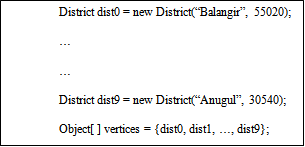The vertices can be objects of any type. For example, you may consider districts as objects that contain the information such as name, population, MLA, etc. Then all the objects are store in an array of object.

POJO CLASS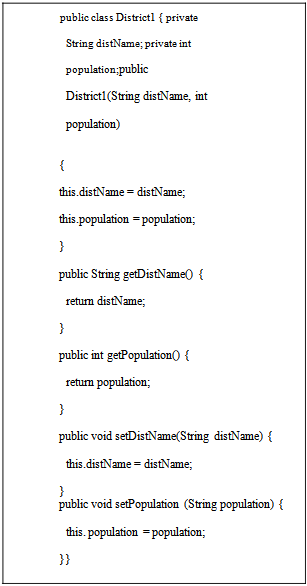Object Graph Controller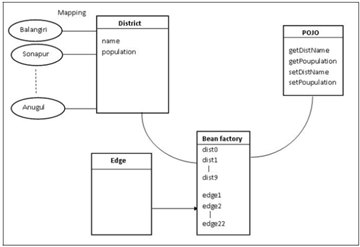All the vertex(district) are map to the District class. In Distance class, name and population are the variable of object in District class. So after mapping all the district name (anugul, sonapur, etc) becomes object of District class and object store in Bean factory. The variable of Edge class is vertex that means District class objects are variable of Edge class. The POJO class contains all the getter, setter method. In BFS class, we found the searching order of vertices.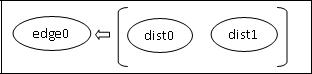This image shows these are two vertex or object in District class, and connecting both form a edge or edge object in Edge class.

3.1.3. Representing Edges
Edge array:

The edge can be represented using a two-dimensional array. For example, to represent the edges in the graph in Figure 1.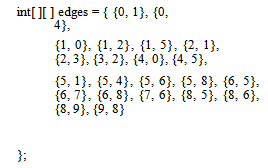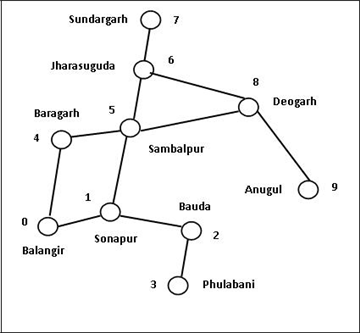List of edge objects:

To represent the edges is to define edges as objects and store the edges in a java.util.ArrayList. For example, to represent the edges in the graph in Figure 1.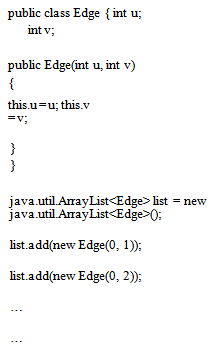### 4. Conclusion

In this paper, the view point of vertex and edge connectivity is introduced. Graph theory is a deep area for programmers. It can be used to solve complex problems. Graph theory is difficult to understand and also for implement. If we implement it by using object oriented approach then it will be easy to implement.

### References

  A.K. Rath, A.K. Jagadev, “Data Structures using C”, 2007.In article  Y. Daniel Liang, “Introduction to Java Programming”, 2010.In article  Tero Harju, “GRAPH THEORY”, University of Turku, Finland, 1994-2011.In article  J. A. Bondy, U. S. Murty, “Graph Theory with Application”, University of Waterloo, Canada, 1976.In article View Article  Keijo Ruohonen, “Graph Theory”, 2013.In article